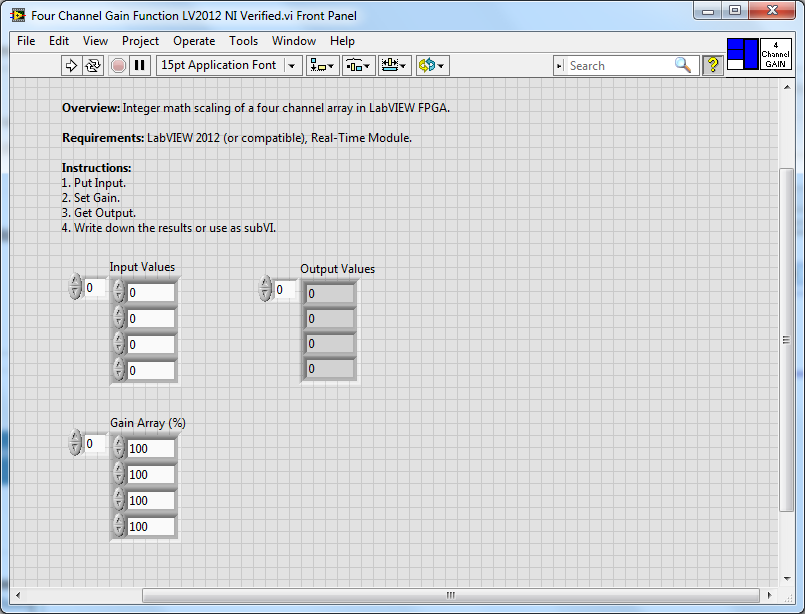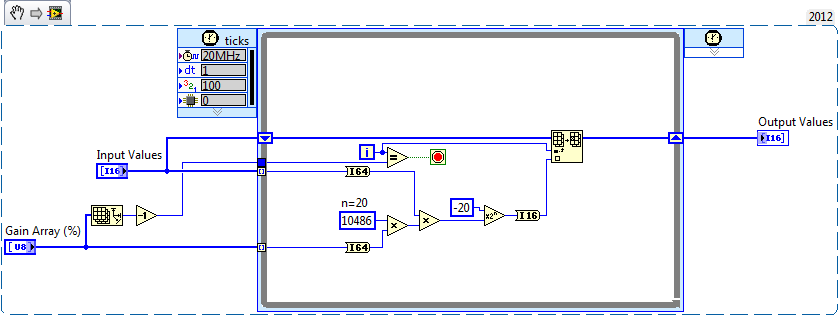# Example Code

## Products and Environment

This section reflects the products and operating system used to create the example.

To download NI software, including the products shown below, visit ni.com/downloads.

### Software

• LabVIEW Real-Time Module
• LabVIEW

## Code and Documents

### Attachment

Overview

Integer math scaling of a four channel array in LabVIEW FPGA.

Description

This example uses integer math to scale an array of four input channels in a LabVIEW FPGA application.  This example will not work within a Single-Cycle Timed Loop, as it uses a Single-Cycle timed loop to index through different channels.Using integer math scaling, the gain value (in percentage) is multiplied by (220)/100 = 10486.  The scaled gain value is then multiplied by the corresponding channel value, and the result is then multiplied by 2(-20).This method of multipling and then dividing by a large power of 2 is a common way of working with integer math in LabVIEW FPGA.

Requirements: LabVIEW 2012 (or compatible), Real-Time Module. Hardware: none.

Steps to Implement or Execute Code:

1. Put Input.
2. Set Gain.
3. Get Output.
4. Write down the results or use as subVI.

Additional Information or References:**This document has been updated to meet the current required format for the NI Code Exchange.**

Example code from the Example Code Exchange in the NI Community is licensed with the MIT license.

Contributors